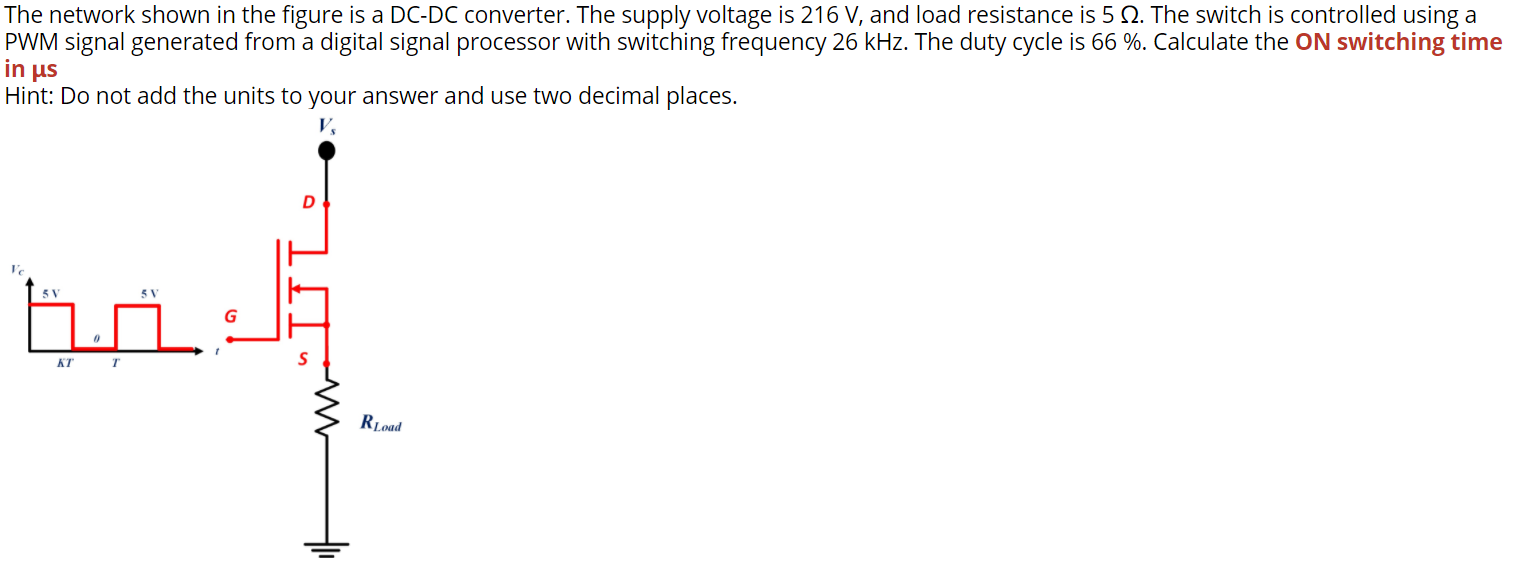# Question The network shown in the figure is a DC-DC converter. The supply voltage is 216 V, and load resistance is 5 12. The switch is controlled using a PWM signal generated from a digital signal processor with switching frequency 26 kHz. The duty cycle is 66 %. Calculate the ON switching time in us Hint: Do not add the units to your answer and use two decimal places. V D 5 V G KT T S RoadMEDKWW The Asker · Electrical EngineeringTranscribed Image Text: The network shown in the figure is a DC-DC converter. The supply voltage is 216 V, and load resistance is 5 12. The switch is controlled using a PWM signal generated from a digital signal processor with switching frequency 26 kHz. The duty cycle is 66 %. Calculate the ON switching time in us Hint: Do not add the units to your answer and use two decimal places. V D 5 V G KT T S Road
More
Transcribed Image Text: The network shown in the figure is a DC-DC converter. The supply voltage is 216 V, and load resistance is 5 12. The switch is controlled using a PWM signal generated from a digital signal processor with switching frequency 26 kHz. The duty cycle is 66 %. Calculate the ON switching time in us Hint: Do not add the units to your answer and use two decimal places. V D 5 V G KT T S Road